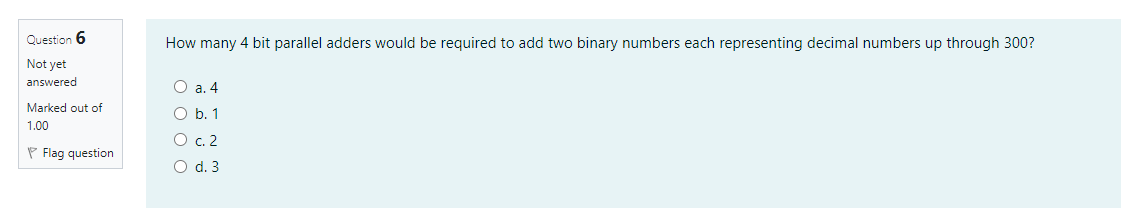# Question Question 6 How many 4 bit parallel adders would be required to add two binary numbers each representing decimal numbers up through 300? Not yet answered O a. 4 Marked out of 1.00 O b. 1 P Flag question O c. 2 O d. 3NKDEUR The Asker · Electrical EngineeringTranscribed Image Text: Question 6 How many 4 bit parallel adders would be required to add two binary numbers each representing decimal numbers up through 300? Not yet answered O a. 4 Marked out of 1.00 O b. 1 P Flag question O c. 2 O d. 3
More
Transcribed Image Text: Question 6 How many 4 bit parallel adders would be required to add two binary numbers each representing decimal numbers up through 300? Not yet answered O a. 4 Marked out of 1.00 O b. 1 P Flag question O c. 2 O d. 3